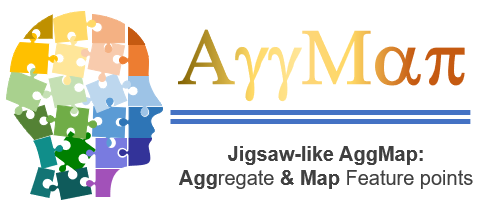# Jigsaw-like aggmap: A Robust and Explainable Multi-Channel Omics Deep Learning Tool

aggmap package is developed to enhance the learning of the unordered and unstructured omics data. aggmap includes theree major modules, they are:

• AggMap: an unsupervised novel feature aggregation tool, which is developed to Aggregate and Map omics features into multi-channel 2D spatial-correlated image-like feature maps (Fmaps) based on their intrinsic correlations.AggMap is an unsupervised learning method because no label is required during feature restructuring. AggMap can be considered as a Fmap jigsaw puzzle solver because it solves jigsaw puzzles of unordered FPs based on their intrinsic similarities and topological structures. It can also be regarded as a representation learning tool because it presents a 1D vector into an image-liked 3D tensor by self-supervised learning. It can employ manifold learning method such as UMAP to restructure unordered FPs by learning their intrinsic structures. The proxy task of umap-based AggMap is to minimize the differences between the two weighted topological graphs built in the input data space and embedding 2D space. Thus, AggMap can expose the overall topology of the FPs to generate structured Fmaps based on the intrinsic structure of FPs.

• AggMapNet: a simple yet efficient CNN-based supervised learning model, which is developed for learning the output structured Fmaps of AggMap .

• Explainers: the model explaination modules (Shapley-explainer and Simply-explainer), which are developed to calculate the local and global feature importance, and based on the 2D-grid of AggMap, we can generate the explaination saliency-map based on the the feature importance score

The details for the theory and usage can be found in our paper on NAR and SSRN :

• Shen W X, Liu Y, Chen Y, et al. AggMapNet: enhanced and explainable low-sample omics deep learning with feature-aggregated multi-channel networks[J]. Nucleic Acids Research, 2022, 50(8): e45-e45.

• Shen, W. X., Liang, S. R., Jiang Y., et al. Enhanced Metagenomic Deep Learning for Disease Prediction and Reproducible Signature Identification by Restructured Microbiome 2D-Representations. SSRN: http://dx.doi.org/10.2139/ssrn.4129422, under review.

You can find the software on github.

Look how easy it is to use:

```import pandas as pd
from aggmap import AggMap, AggMapNet

dfx = pd.DataFrame(data.data, columns=data.feature_names)
dfy = pd.get_dummies(pd.Series(data.target))

# AggMap object definition, fitting, and saving
mp = AggMap(dfx, metric = 'correlation')
mp.fit(cluster_channels=5, emb_method = 'umap', verbose=0)
mp.save('agg.mp')

# AggMap visulizations: Hierarchical tree, embeddng scatter and grid
mp.plot_tree()
mp.plot_grid(enabled_data_labels=True)

# Transoformation of 1d vectors to 3D Fmaps (-1, w, h, c) by AggMap
X = mp.batch_transform(dfx.values, n_jobs=4, scale_method = 'minmax')
y = dfy.values

# AggMapNet training, validation, early stopping, and saving
clf = AggMapNet.MultiClassEstimator(epochs=50, gpuid=0)
clf.fit(X, y, X_valid=None, y_valid=None)
clf.save_model('agg.model')

# Model explaination by simply-explainer: global, local
simp_explainer = AggMapNet.simply_explainer(clf, mp)
global_simp_importance = simp_explainer.global_explain(clf.X_, clf.y_)
local_simp_importance = simp_explainer.local_explain(clf.X_[], clf.y_[])

# Model explaination by shapley-explainer: global, local
shap_explainer = AggMapNet.shapley_explainer(clf, mp)
global_shap_importance = shap_explainer.global_explain(clf.X_)
local_shap_importance = shap_explainer.local_explain(clf.X_[])
```

## Contribute

• Issue Tracker: https//github.com/shenwanxiang/bidd-aggmap/issues

• Source Code: https//github.com/shenwanxiang/bidd-aggmap/

## Support

If you are having issues, please let us know. We have a mailing list located at: wanxiang.shen@u.nus.edu